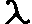#Computer Algebra & Symbolic Computation

Format: A heirarchical expression formatter for Macsyma.
This package provides facilities for user-directed hierarchical structuring of expressions, as well as for directing simplifications to selected subexpressions. It emphasizes a semantic rather than syntactic description of the desired form. The package also provides utilities for obtaining efficiently the coefficients of polynomials, trigonometric sums and power series.
Download format.tar.gz, the tar'ed and gzipped package. Some examples may be clearer than words:
```/* A Basic rearrangement: */
(c1) format((a+b*x)*(c-x)^2,%poly(x),%factor);
3                2                        2
(d1) b x  - (2 b c - a) x  + c (b c - 2 a) x + a c

/* A `mathematical' alternative to map or substpart...*/
(c2) format((1+2*a+a^2)*b + a*(1+2*b+b^2),%sum,%product,%factor);
2          2
(d2) a (b + 1)  + (a + 1)  b

/* Focussing simplifications */
(c3) foo:X^2*SIN(Y)^4-2*X^2*SIN(Y)^2+X^4*COS(Y)^4-2*X^4*COS(Y)^2+X^4+X^2+1\$

(c4) trigsimp(foo);
4    2     4         4    2       4
(d4) (x  + x ) cos (y) - 2 x  cos (y) + x  + 1

/* That helps, but this is better: */
(c5) format(foo,%poly(x),trigsimp);
4    4       2    4
(d5) x  sin (y) + x  cos (y) + 1
```
The package is written in Lisp, but should run under DOE & Macsyma Inc's versions of Macsyma. A similar package has been partially implemented for Maple; if there is sufficient interest, I may complete it.

## Other Resources: Math, Symbolic Computation, Computer Algebra

Created by Bruce Miller @ NIST (bruce.miller@nist.gov) Disclaimer.